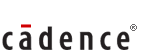Posted by :
Vimala
(11)

1. Inorder and preorder trees (expressions) are given and post order tree (expression) is to be found out.

2. int v,u;
while(v != 0)
{
t = v % u;
v = u;
u = t;
}
find the time complexity of the above program.

3. x is passed by reference, y passed by value.
x = 3, y = 2;
foo(x, y)
var integer x, y;
{
x = x + 2;
y = y + 3;
}
main()
{
x = 5;
y = 5;
foo(x, y);
print (x, y);
}
output of the above pseudo code.

4. given a grammar, in which some productions of if then else etc were given. you had to choose one option that can be derived out of the grammar.

5. how many flip flops you require for modulo 19 counter.

6. ring counter\'s initial state is 01000. after how many clock cylces will it return to the initial state.

7. some boolesn expression of the form x\'y\'z\' + yz + .. ( something like this) find the simplified expression

8. given 6 bit mantissa in 2s complement form and 4 bit exponent is in excess-4 form in a floating point representation, find the number ans -(something) * ( 2 to the power 3)

9. A signed no is stored in 10-bit register, what is the max and min possible value of the number.
A few apti qs are follows.

10. A room is 30 X 12 X 12. a spider is ont the middle of the samller wall, 1 feet from the top, and a fly is ont he middle of the opposite wall 1 feet from the bottom. what is the min distance reqd for the spider to crawl to the fly.

11. A man while going dowm in a escalator(which is miving down) takes 50 steps to reach down and while going up takes 125 steps. If he goes 5 times faster upwards than downwards. What will be the total no of steps if the escalator werent moving.

12. 2/3 of corckery(plates) are broken, 1/2 have someother thing(handle) broken , 1/4 are both broken and handle broken. Ultimately only 2 pieces of corckery were without any defect. How many crockery were there in total.

13. It is difficult to draw a figure but another question was in which some NAND and OR gates were given. ans - Z = true. cadence parer...
As I wrote you 75 Marks paper..time limit 1.15 H